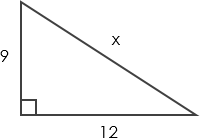# Pythagoras Theorem Calculator

This is an online Pythagoras Theorem calculator that helps you find out the length of any side of a right triangle, provided you know the lengths of the other two sides. Besides the calculator, it also provides you with information about Pythagoras Theorem.

## What is Pythagoras Theorem?

The Pythagorean Theorem (aka. Pythagoras’ theorem) describes the relations between the three sides of a right triangle. It states that the sum of the squares of the shorter sides of a right triangle equals the square of the hypotenuse.  Here is the formula:

`a2 + b2 = c2`

where a and b are the length of the two shorter sides and c is the length of the hypotenuse.

The theorem was named after the ancient Greek philosopher and mathematician Pythagoras, who lived in the sixth century BC.

## Pythagoras Theorem example

Let’s solve the triangle below. Find x.The triangle is a right-angled triangle. Therefore, we can find the length x using the Pythagoras Theorem.

x is the hypotenuse. We first start by writing the equation: `92 + 122 = x2`

We can then use algebra to find x:

92 + 122 = x2

225 = `x2`

x = 15

## How to use the Pythagoras Theorem calculator?

1. Under the Find box, select the side that you want to find out (a / b / c).
2. Input the length of the two sides that you have. For example, suppose you want to find c, enter the value of a and b.
3. The result is automatically calculated and displayed in the field below.

#### This calculator is created with

the best online spreadsheet editor with excellent formula and editing capability.

For more calculators for finance, mathematics health, unit converters and more check out our calculators collection.